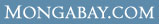WHAT ARE THE AVERAGE Wages & salaries for Computer and mathematical occupations in Lawrence, KS ?

 Overview Every year U.S. Department of Labor's Bureau of Labor Statistics releases employment and wage figures. This data was released in May 2006 and covers Wages & salaries for Computer and mathematical occupations in Lawrence, KS Computer and mathematical occupations (Occupation [OCC] code: 15-0000) in Lawrence, KS (BLS Area code: 29940 ) earned an average wage of \$26.40 per hour and an average annual salary of \$54,910 The BLS estimates that in Lawrence, KS there were around 1,190 employed in the field of Computer and mathematical occupations.

Industry Comparison
Industry comparison
Computer and mathematical occupations in Lawrence, KS earned 21% less than average for Computer and mathematical occupations in the United States.

Region/area/city comparison
Computer and mathematical occupations in Lawrence, KS earned 66% more than the average worker in Lawrence, KS.

State salary comparison
Computer and mathematical occupations in Lawrence, KS earned 57% more than the average worker in Kansas.

National salary comparison
Computer and mathematical occupations in Lawrence, KS earned 40% more than the average worker in the United States.

Wage and salary details
More detailed employment information for Computer and mathematical occupations in Lawrence, KS

Hourly wage data for Computer and mathematical occupations in Lawrence, KS

 Mean hourly wage: \$26.40 Tenth (10th) percentile hourly wage: \$13.50 Twenty-fifth (25th) percentile hourly wage: \$17.65 Fiftieth (50th) percentile wage [median] hourly wage: \$24.01 Seventy-fifth (75th) percentile hourly wage: \$34.04 Ninetieth (90th) percentile hourly wage: \$44.10

Salary figures for Computer and mathematical occupations in Lawrence, KS

 Mean annual salary: \$54,910 Tenth (10th) percentile annual salary: \$28,080 Twenty-fifth (25th) percentile annual salary: \$36,700 Fiftieth (50th) percentile wage [median] annual salary: \$49,950 Seventy-fifth (75th) percentile annual salary: \$70,810 Ninetieth (90th) percentile annual salary: \$91,730

Indexwhat's new | rainforests home | help support the site | madagascar | search | about | contact